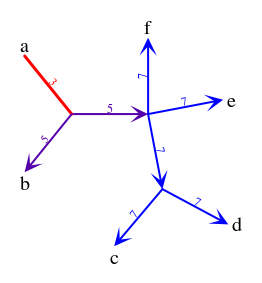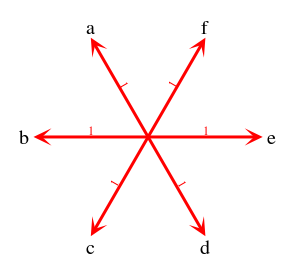## Robinson-Foulds - unrooted gene trees vs rooted species trees

Contents

General description
This page contains complementary material for the article : prototype software and results of experiments.

Program
Program rf in package rf.tgz have the following options:
rf [h] [-g FILE|-] [-s FILE|-] [FILE1] [FILE2] ...
FILE can be: - (minus) - stdin, filename or a string (if file of given name does not exists). Option -g defines gene trees, option -s species trees. Trees are defined in standard nested parenthesis notation. If no option is used rf assumes that the first tree is a gene tree, then a species tree, a gene tree, etc.

Output. Nodes are decorated with atributes:
cost=NUM (total cost of rooting placed on the edge),
top=NUM (direction of edge: 0=empty ===, -1=single <===, 1=single ===>, 2=double <===>). See  for more details.

Example 1.
rf.py -g "((a,b),(c,d),e,f)" -s "(a,(b,(c,(d,(e,f)))))"
(((a top=0 cost=3,b top=-1 cost=5) top=1 cost=5,(c top=-1 cost=7,d top=-1 cost=7) top=-1 cost=7,e top=-1 cost=7) top=1 cost=7,f top=-1 cost=7)Example 2.
rf.py -g "(a,b,c,d,e,f)" -s "(a,b,c,d,e,f)"
((a top=-1 cost=1,b top=-1 cost=1,c top=-1 cost=1,d top=-1 cost=1,e top=-1 cost=1) top=1 cost=1,f top=-1 cost=1)All files can be accessed from this directory: files.
• rf.tgz - linux binaries generated from python source by pyinstaller; run rf -h program to get help
• phymltres.tgz - 3150 unrooted gene family trees from OrthoMCL-DB 5.0 database
• treerepr.tgz - all results of RF analysis:
• FAMIDgt.txt - simplified gene trees
• FAMIDgtflat.txt - flattened gene tree
• FAMIDstar.txt - RF cost and star-like topology (top=1 arrow up, top=-1 arrow down, top=0 - single edge, top=2 double edge) with costs;
• FAMIDstcontr.txt - contracted species tree
• stars.tgz - star-like topologies per each gene family (jpeg's)
• ncbishort.tree - species tree (rooted) based on NCBI taxomony

Bibliography
 Pawel Gorecki and Oliver Eulenstein, A Robinson-Foulds measure to compare unrooted trees with rooted trees

Homepage
 Last update: Feb. 9, 2012